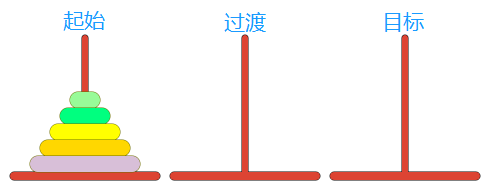# Python实现 — — 汉诺塔问题``````count=0
def hanoi(n,a,b,c):
global count
if n == 1 :
print("{}:{}->{}".format(1,a,c))
count+=1
else:
hanoi(n-1,a,c,b)
print("{}:{}->{}".format(n,a,c))
hanoi(n-1,b,a,c)
count+=1

hanoi(2,"A","B","C")
print(count)``````（1）该怎么搬运

（2）需要多少步

（1）搬运的圆盘是第几层的

（2）从哪根柱子搬运到哪根柱子

``````def hanoi(n,a,b,c):
if n == 1:
print("{}:{}->{}".format(1,a,c))
else:
hanoi(n-1,a,c,b)
print("{}:{}->{}".format(n,a,c))
hanoi(n-1,b,a,c)

hanoi(2,"A","B","C")``````

OK，我们缩减完代码后下一步就要将这个问题抽象，现在我们知道了题目，但怎么解呢？我们把这个解分为三步：

（1）将上面的n-1个圆盘，从A借助C移动到B

（2）将最下面的一个圆盘，从A移动到C

（3）将上面的n-1个圆盘，从B借助A移动到C

（n-1代表除了最下面一个圆盘的所有圆盘，现在不理解没关系后面有解释，现在只需要记住就行了）